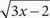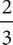# SAT Math Multiple Choice Question 45: Answer and Explanation

### Test Information

Question: 45

1. If f (x) =, what is the smallest possible value of f (x) ?

• A. 0
• B.• C. 1
• D. 2

A On this question you can use Plugging In the Answers. The numbers in the answer choices replace the f(x) portion of the equation, so you can just write out the rest of it,, next to each to see if it can be true. Start with (A) since you are looking for the smallest value of f(x). If 0 =, then 0 = 3x - 2 when you square both sides. Add 2 to both sides to get 2 = 3x, and then divide both sides by 3. You get x =. Since this is a real value, the equation works, so the smallest value of f(x) is 0. Choice (A) is correct.